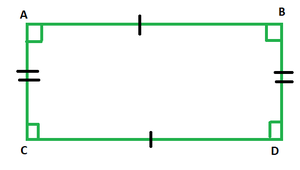GeeksforGeeks App
Open AppBrowser
Continue

# Surface Area of a Rectangle Formula

Geometry includes the study of a plethora of shapes in mathematics. Not only that, their dimensions and numerous calculations pertaining to their dimensions are also done under geometry.

A quadrilateral whose opposite sides are of equal lengths and all the four angles are right angles is called a rectangle. The figure given below depicts a rectangle. Observe how the opposite sides are equal to each- other and all the angles are 90°.### Area of a Rectangle

The area of a rectangle is given as:

A = l × b

where,

• l is the length of the rectangle
• b is the breadth of the rectangle

### Sample Problems

Question 1. What would be the area of the rectangle whose length is 4 m and width is 9 m.

Solution:

Given: l = 4 m, b = 9 m

A = l x b

= 4 x 9

A = 36 m2

Question 2. Find the length of a rectangle if its area is 400 sq. units and width is 50 units.

Solution:

Given: A = 400 sq. units, b = 50 units

A = l x b

400 = l x 50

l = 400/50

= 8 units

Question 3. Find the width of a rectangle whose area and length are 100 sq. units and 25 units respectively.

Solution:

Given: A = 100 sq. units, l = 25 units

A = l x b

100 = 25 x b

b = 100/25

= 4 units

Question 4. What would be the area of the rectangle whose length is 10 m and width is 9 m?

Solution:

Given: l = 10 m, b = 9 m

A = l x b

= 10 x 9

A = 90 m2

Question 5. What would be the area of the rectangle whose length is 34 m and width is 39 m?

Solution:

Given: l = 34 m, b = 39 m

A = l x b

= 34 x 39

A = 1326 m2

My Personal Notes arrow_drop_up
Related Tutorials